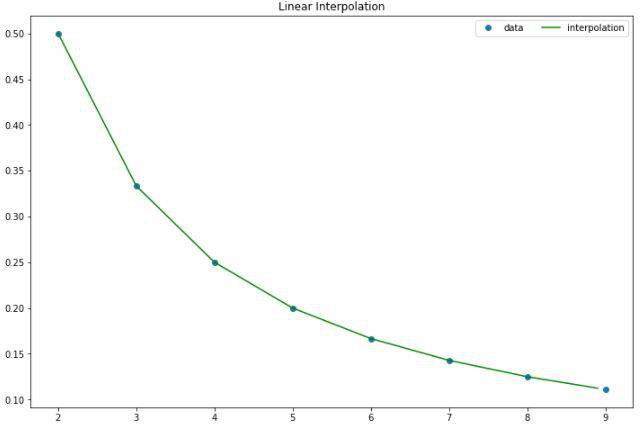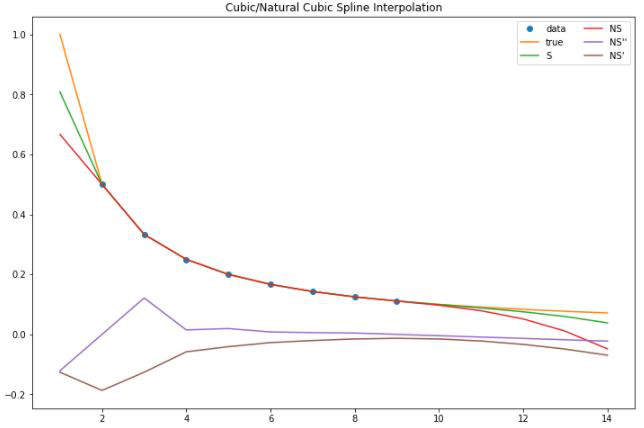GeeksforGeeks App
Open AppBrowser
Continue

# Natural Cubic spline

### Cubic Spline:

The cubic spline is a spline that uses the third-degree polynomial which satisfied the given m control points. To derive the solutions for the cubic spline, we assume the second derivation 0 at endpoints, which in turn provides a boundary condition that adds two equations to m-2 equations to make them solvable. The system of equations for the Cubic spline for 1-dimension can be given by:We take a set of points [xi, yi] for i = 0, 1, …, n for the function y = f(x). The cubic spline interpolation is a piecewise continuous curve, passing through each of the values in the table.

• Following are the conditions for the spline of degree K=3:
• The domain of s is in intervals of [a, b].
• S, S’, S” are all continuous function on [a,b].Here Si(x) is the cubic polynomial that will be used on the subinterval [xi, xi+1]

Since, there are n intervals and 4 coefficients of each equation, for that we require a 4n parameters to solve the spline, we can get 2n equation from the fact that each of the cubic spline equation should satisfy the value at both ends:The above cubic spline equations should not only continuous and differentiable but also have defined first and second derivatives that are also continuous on control points.andFor 1, 2, 3…n-1 provides the 2n -2 equation constraints. So, we need 2 more equations to solve the above cubic spline. For that, we will use some natural boundary conditions.

### Natural Cubic Spline:

In Natural cubic spline, we assume that the second derivative of the spline at boundary points is 0:Now, since the S(x) is a third-order polynomial we know that S”(x) is a linear spline which interpolates. Hence, first, we construct S”(x) then integrate it twice to obtain S(x).

Now, let’s assume t_i = x_i for i= 0, 1,…n, andand from the natural boundary condition. Twice differentiating a cubic spline gives a linear spline which can be written as:where,Now, the equation becomes:Integrating this equation two times to obtain the cubic spline:where,Now, to check for the continuity of derivative at t_i i.e S^{‘}_{i}(t_i) = S^{‘}_{i-1}(t_{i}). We first need to find derivatives and put that condition:where,Putting the above equation for the continuity and solving it gives the following equation:Take, v_i = 6(b_i – b_{i-1}) and the above equation can be written as form of matrix:## Implementation

• In this implementation, we will be performing the spline interpolation for function f(x) = 1/x for points b/w 2-10 with cubic spline that satisfied natural boundary condition.

## Python3

 #importsimport matplotlib.pyplot as pltimport numpy as npfrom scipy.interpolate import CubicSpline, interp1dplt.rcParams['figure.figsize'] =(12,8)  x = np.arange(2,10)y = 1/(x)# apply cubic spline interpolationcs = CubicSpline(x, y, extrapolate=True)# apply natural cubic spline interpolationns = CubicSpline(x, y,bc_type='natural', extrapolate=True)  # Apply Linear interpolationlinear_int = interp1d(x,y)  xs = np.arange(2, 9, 0.1)ys = linear_int(xs)  # plot linear interpolationplt.plot(x, y,'o', label='data')plt.plot(xs,ys,  label="interpolation", color='green')plt.legend(loc='upper right', ncol=2)plt.title('Linear Interpolation')plt.show()  # define a new xsxs = np.arange(1,15)#plot cubic spline and natural cubic splineplt.plot(x, y, 'o', label='data')plt.plot(xs, 1/(xs), label='true')plt.plot(xs, cs(xs), label="S")plt.plot(xs, ns(xs), label="NS")plt.plot(xs, ns(xs,2), label="NS''")plt.plot(xs, ns(xs,1), label="NS'")  plt.legend(loc='upper right', ncol=2)plt.title('Cubic/Natural Cubic Spline Interpolation')plt.show()  # check for boundary conditionprint("Value of double differentiation at boundary conditions are %s and %s"      %(ns(2,2),ns(10,2)))

Output:

Value of double differentiation at boundary conditions are -1.1102230246251565e-16 and -0.00450262550915248Linear InterpolationCubic/Natural Spline Interpolation

### References:

My Personal Notes arrow_drop_up